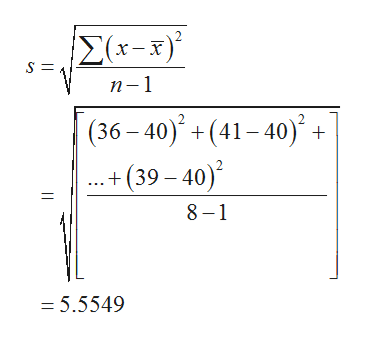# Construct a 90% confidence interval for the mean highway mileage for hybrid cars. A random sample of 8 hybrid cars had the following highway mileages in mpg.36 41 37 45 50 40 32 39

Question
1 views

Construct a 90% confidence interval for the mean highway mileage for hybrid cars. A random sample of 8 hybrid cars had the following highway mileages in mpg.
36 41 37 45 50 40 32 39

check_circle

Step 1

Solution:

The sample mean is obtained below:

From the given information, number of observations is 8 and confidence level is 90%.

The required mean is,

Step 2

The sample standard deviation is obtained below:help_outlineImage TranscriptioncloseE(x-x) п-1 (36 – 40) + (41– 40)° + .+ (39 – 40) 8 – 1 = 5.5549 fullscreen
Step 3

The critical value is obtained below:

The level of significance=1-0.90=0.10.

The degrees of freedom= 8-1=7

The critical value is,

From the EXCEL, using the formula, =T.INV.2T(0.10,7), the critical value is...

### Want to see the full answer?

See Solution

#### Want to see this answer and more?

Solutions are written by subject experts who are available 24/7. Questions are typically answered within 1 hour.*

See Solution
*Response times may vary by subject and question.
Tagged in

### Hypothesis Testing